Latest Banking jobs   »   Quantitative Aptitude Quiz For RRB PO...   »   Quantitative Aptitude Quiz For RRB PO...

# Quantitative Aptitude Quiz For RRB PO Mains 2021- 19th September

Directions (1 – 2): The following questions are accompanied by two statements (I) and (II). You have to determine which statements(s) is/are sufficient/necessary to answer the questions.
(a) Statement (I) alone is sufficient to answer the question but statement (II) alone is not sufficient to answer the questions.
(b) Statement (II) alone is sufficient to answer the question but statement (I) alone is not sufficient to answer the question.
(c) Both the statements taken together are necessary to answer the questions, but neither of the statements alone is sufficient to answer the question.
(d) Either statement (I) or statement (II) by itself is sufficient to answer the question.
(e) Statements (I) and (II) taken together are not sufficient to answer the question.

Q1. What is the population of the city A?
I. The ratio of the population of males and females in city A is 27 : 23 and the difference between their population is 100000.
II. The population of city A is 80% of that of city B. The difference between populations of city A and city B is 312500.

Q2. What is the volume of the sphere?
I. Curved surface area of hemisphere is equal to the total surface area of the cylinder having radius and height in ratio 3 : 4.
II. When we cut sphere into two hemispheres then total surface area is equal to the area of a circle whose radius is 21 cm.

Directions (3-5): The following questions are accompanied by two statements (I) and (II). You have to determine which statements(s) is/are sufficient/necessary to answer the questions.
(a) Statement (I) alone is sufficient to answer the question but statement (II) alone is not sufficient to answer the questions.
(b) Statement (II) alone is sufficient to answer the question but statement (I) alone is not sufficient to answer the question.
(c) Both the statements taken together are necessary to answer the questions, but neither of the statements alone is sufficient to answer the question.
(d) Either statement (I) or statement (II) by itself is sufficient to answer the question.
(e) Statements (I) and (II) taken together are not sufficient to answer the question.

Q3. What is total surface area of cone?
I .Radius of cone is 4 cm less than side of square, which area is 324 cm2.
II .Height of cone is 3 cm more than radius of a circle, whose circumference is 44 cm.

Q4. What is rate of interest?
I . A man invested an amount for four years on simple interest and gets a total amount, which is 160% of invested amount.
II . Amir invested Rs. 8800 on simple interest and gets a total interest of Rs. 3960 after three years.

Q5. What is base of a triangle ABC which has AB is its base?
I . Height and base of triangle in the ratio of 3 : 2.
II . Area of triangle is 120 cm2 and perimeter of triangle is 80 cm and height is half of the perimeter.

Directions (6-7): In the following questions three statements either A, B and C or I, II and III are given. You have to use your knowledge of mathematics to answer which statement(s) is/are sufficient to answer the question.

Q6. What is the age of Ravi?
A: Four years ago, Raju was as old as Ravi is at present.
B: Rita’s present age is two times of Raju’s present age.
C: The average age of Ravi and Rita is 19 years.
(a) A is sufficient to give answer
(b) Any two statements are sufficient to give answer
(c) All of three statements are necessary to give answer
(d) Even using all the three statements answer cannot be found.
(e) Only C is sufficient.

Q7. What is the cost price of wrist watch?
A: Shopkeeper gives 20% discount on a speaker.
B: The marked price of wrist watch is 25% more than that of speaker.
C: The shopkeeper earns a profit of 10% after selling the speaker.
(a) Any two of them are sufficient
(b) All the three statements are required
(c) Only C is sufficient.
(d) Even using all statements, answer cannot be found
(e) Only A is sufficient

Directions (8-10): In each of these questions, two equations are given. You have to solve both the equations and give answer
(a) if x>y
(b) if x≥y
(c) if x<y
(d) if x ≤y
(e) if x = y or no relation can be established between x and y.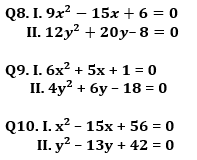Solutions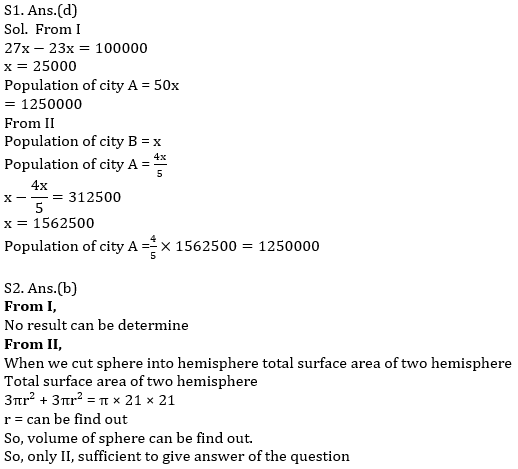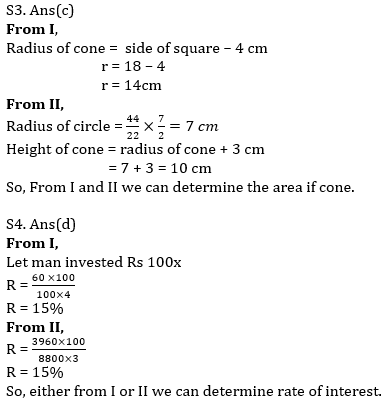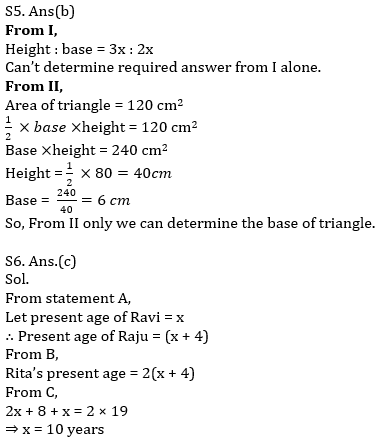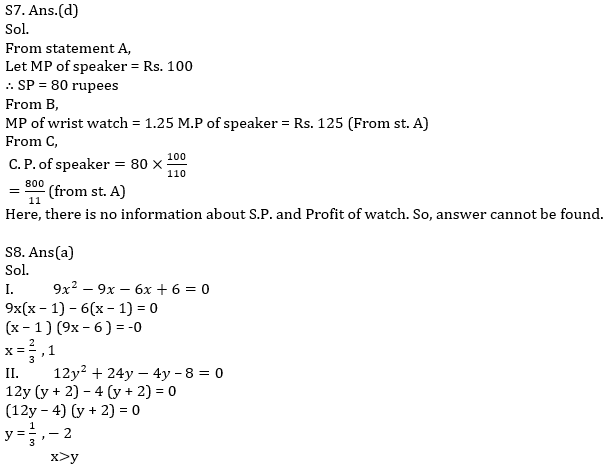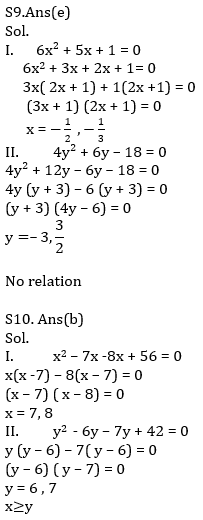Click Here to Register for Bank Exams 2021 Preparation Material#### Congratulations!Download Hindu Review of October 2021: Free PDF